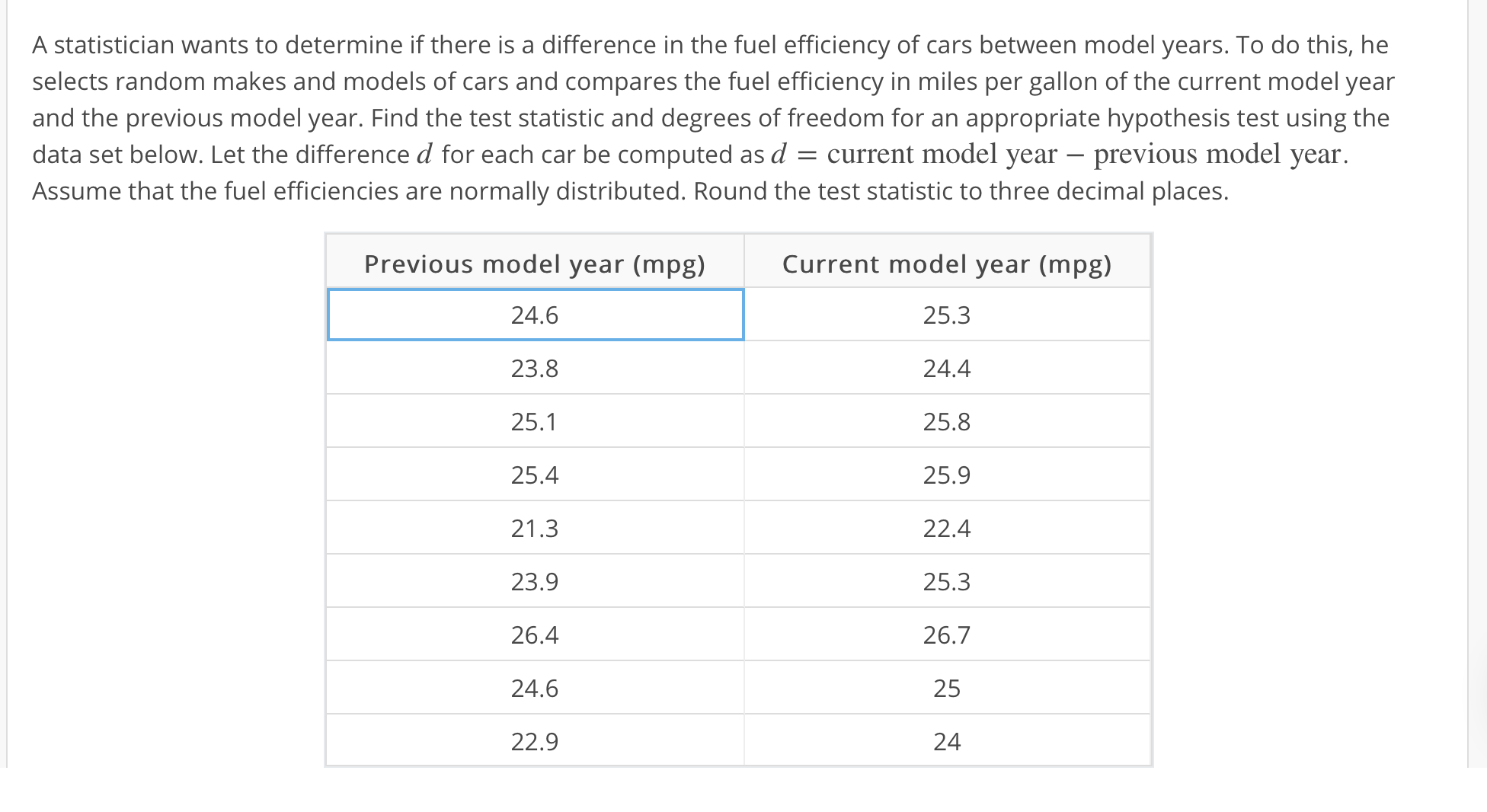# A statistician wants to determine if there is a difference in the fuel efficiency of cars between model years. To do this, heselects random makes and models of cars and compares the fuel efficiency in miles per gallon of the current model yearand the previous model year. Find the test statistic and degrees of freedom for an appropriate hypothesis test using thedata set below. Let the difference ₫ for each car be computed as ₫ current model year-previous model yearAssume that the fuel efficiencies are normally distributed. Round the test statistic to three decimal places.Previous model year (mpg)24.623.825.125.421.323.926.424.622.9Current model year (mpg)25.324.425.825.922.425.326.72524

Question
13 views

T= , df=help_outlineImage TranscriptioncloseA statistician wants to determine if there is a difference in the fuel efficiency of cars between model years. To do this, he selects random makes and models of cars and compares the fuel efficiency in miles per gallon of the current model year and the previous model year. Find the test statistic and degrees of freedom for an appropriate hypothesis test using the data set below. Let the difference ₫ for each car be computed as ₫ current model year-previous model year Assume that the fuel efficiencies are normally distributed. Round the test statistic to three decimal places. Previous model year (mpg) 24.6 23.8 25.1 25.4 21.3 23.9 26.4 24.6 22.9 Current model year (mpg) 25.3 24.4 25.8 25.9 22.4 25.3 26.7 25 24 fullscreen
check_circle

Step 1

Paired t-test statistic:

In order to test a hypothesis regarding whether the difference between a paired set of n observations (xi, yi) is significant or not, the paired t-test is used. The test statistic for the paired t-test is given as follows:

Step 2

Calculation:

The following table shows the necessary calculations for finding the test statistic:

Step 3

Further calculations:...

### Want to see the full answer?

See Solution

#### Want to see this answer and more?

Solutions are written by subject experts who are available 24/7. Questions are typically answered within 1 hour.*

See Solution
*Response times may vary by subject and question.
Tagged in

### Data